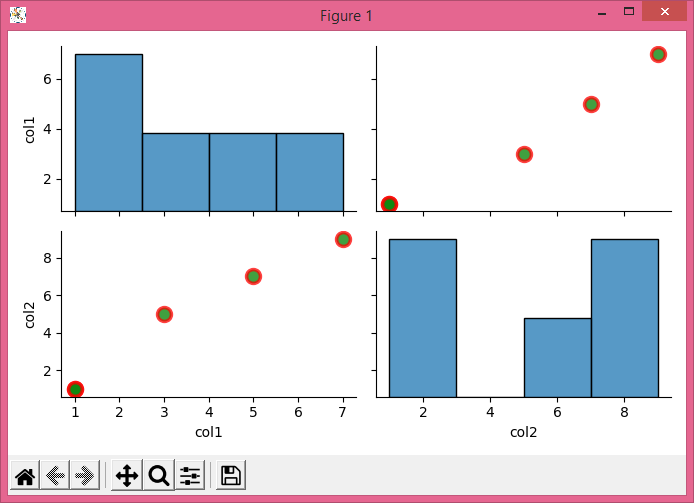# How to adjust transparency (alpha) in Seaborn pairplot using Matplotlib?

To adjust transparency, i.e., aplha in Seaborn pairplot, we can change the value of alpha.

## Steps

• Create a dataframe using Pandas with two keys, col1 and col2.

• Initialize the variable, alpha, for transparency.

• Use pairplot() method to plot pairwise relationships in a dataset. Use df (from step 1), kind="scatter", and set the plot size, edgecolor, facecolor, linewidth and alpha vaues in the arguments.

• To display the figure, use show() method.

## Example

import pandas as pd
import seaborn as sns
from matplotlib import pyplot as plt
plt.rcParams["figure.figsize"] = [7.00, 3.50]
plt.rcParams["figure.autolayout"] = True
df = pd.DataFrame({"col1": [1, 3, 5, 7, 1], "col2": [1, 5, 7, 9, 1]})
alpha = 0.75
sns.pairplot(df, kind="scatter", plot_kws=dict(s=100, edgecolor="red",
fc='green', linewidth=2.5, alpha=alpha))
plt.show()

## Output